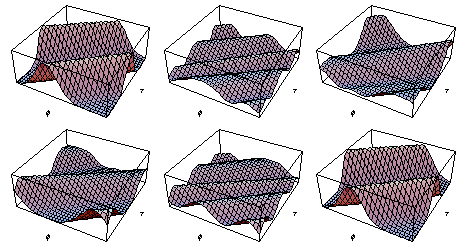# Particle moving on a ringCH352 Physical Chemistry Fall 2002Updated Friday, October 24, 2003 4:48 PM

Department of Chemistry, Boston University, Boston MA 02215Here is an animation probability density of a quantum particle moving on a ring. The particle is described by a wavefunction composed of five m values: 8, 9, 10, 11, and 12. The relative weights of the m values fall off symmetrically from m = 10.The motion covers one period of evolution of the ring. This period is 2 pi time units. One time unit is equal the hbar/(2 m r^2), where m is the mass of the particle and r is the radius of the ring. For an HCl molecule the time unit is 5 x 10^-13 seconds.